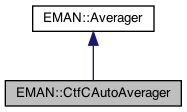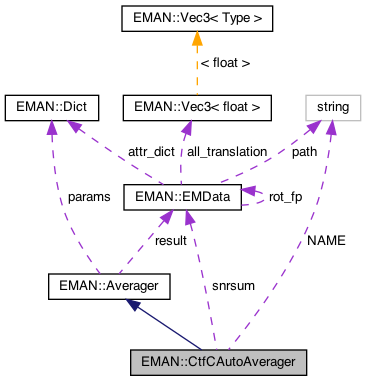EMAN2
EMAN::CtfCAutoAverager Class Reference

CtfCWautoAverager averages the images with CTF correction with a Wiener filter. More...

`#include <averager.h>`

Inheritance diagram for EMAN::CtfCAutoAverager:[legend]
Collaboration diagram for EMAN::CtfCAutoAverager:[legend]

## Public Member Functions

CtfCAutoAverager ()

To add an image to the Averager. More...

EMDatafinish ()
Finish up the averaging and return the result. More...

string get_name () const
Get the Averager's name. More...

string get_desc () const

void set_params (const Dict &new_params)
Set the Averager parameters using a key/value dictionary. More...Public Member Functions inherited from EMAN::Averager
Averager ()

virtual ~Averager ()

virtual void add_image_list (const vector< EMData * > &images)
To add multiple images to the Averager. More...

virtual void mult (const float &s)
Multiply the result image by some floating point constant This is useful when weighting the input images prior to calling add_image - a situation where it is likely you want to divide by the sum of the weights. More...

virtual TypeDict get_param_types () const
Get Averager parameter information in a dictionary. More...

## Static Public Member Functions

static AveragerNEW ()

## Static Public Attributes

static const string NAME = "ctf.auto"

## Protected Attributes

EMDatasnrsum

int nimgProtected Attributes inherited from EMAN::Averager
Dict params

EMDataresult

## Detailed Description

CtfCWautoAverager averages the images with CTF correction with a Wiener filter.

The Weiner filter is estimated directly from the data.

Definition at line 565 of file averager.h.

## ◆ CtfCAutoAverager()

 CtfCAutoAverager::CtfCAutoAverager ( )

Definition at line 1131 of file averager.cpp.

1132 : nimg(0)
1133{
1134
1135}

Referenced by NEW().

## Member Function Documentation

 void CtfCAutoAverager::add_image ( EMData * image )
virtual

To add an image to the Averager.

This image will be averaged in this function.

Parameters
 image The image to be averaged.

Reimplemented from EMAN::Averager.

Definition at line 1138 of file averager.cpp.

1139{
1140 if (!image) {
1141 return;
1142 }
1143
1144
1145
1146 EMData *fft=image->do_fft();
1147
1148 if (nimg >= 1 && !EMUtil::is_same_size(fft, result)) {
1149 LOGERR("%s Averager can only process images of the same size", get_name().c_str());
1150 return;
1151 }
1152
1153 nimg++;
1154 if (nimg == 1) {
1156 result->to_zero();
1157 }
1158
1159 Ctf *ctf = (Ctf *)image->get_attr("ctf");
1160 float b=ctf->bfactor;
1161 ctf->bfactor=0; // NO B-FACTOR CORRECTION !
1162
1163 EMData *snr = result -> copy();
1165 EMData *ctfi = result-> copy();
1167
1169
1170 float *outd = result->get_data();
1171 float *ind = fft->get_data();
1172 float *snrd = snr->get_data();
1173 float *ctfd = ctfi->get_data();
1174
1175 size_t sz=snr->get_xsize()*snr->get_ysize();
1176 for (size_t i = 0; i < sz; i+=2) {
1177 if (snrd[i]<=0) snrd[i]=0.001; // used to be 0. Trying to insure that there is always at least a little signal used. In cases with dense particles, SNR may be dramatically underestimated
1178 ctfd[i]=fabs(ctfd[i]);
1179
1180 // This limits the maximum possible amplification in CTF correction to 10x
1181 if (ctfd[i]<.05) ctfd[i]=0.05f;
1182// {
1183// if (snrd[i]<=0) ctfd[i]=.05f;
1184// else ctfd[i]=snrd[i]*10.0f;
1185// }
1186
1187 // SNR weight and CTF correction
1188 outd[i]+=ind[i]*snrd[i]/ctfd[i];
1189 outd[i+1]+=ind[i+1]*snrd[i]/ctfd[i];
1190 }
1191
1192 if (nimg==1) {
1194 float *ssnrd=snrsum->get_data();
1195 // we're only using the real component, for Wiener filter we put 1.0 in R, but for just SNR weight we use 0
1196 for (size_t i = 0; i < sz; i+=2) { ssnrd[i]=0.0; ssnrd[i+1]=0.0; }
1197 }
1198 snr->process_inplace("math.absvalue");
1200
1201 delete ctf;
1202 delete fft;
1203 delete snr;
1204 delete ctfi;
1205}
EMData * result
Definition: averager.h:158
string get_name() const
Get the Averager's name.
Definition: averager.h:573
Ctf is the base class for all CTF model.
Definition: ctf.h:60
virtual void compute_2d_complex(EMData *img, CtfType t, XYData *struct_factor=0)=0
float bfactor
Definition: ctf.h:85
@ CTF_AMP
Definition: ctf.h:65
@ CTF_SNR
Definition: ctf.h:68
EMData stores an image's data and defines core image processing routines.
Definition: emdata.h:82
static bool is_same_size(const EMData *image1, const EMData *image2)
Check whether two EMData images are of the same size.
Definition: emutil.cpp:1224
EMData * copy() const
This file is a part of "emdata.h", to use functions in this file, you should "#include "emdata....
#define LOGERR
Definition: log.h:51

## ◆ finish()

 EMData * CtfCAutoAverager::finish ( )
virtual

Finish up the averaging and return the result.

Returns
The averaged image.

Implements EMAN::Averager.

Definition at line 1207 of file averager.cpp.

1208{
1209/* EMData *tmp=result->do_ift();
1210 tmp->write_image("ctfcw.hdf",0);
1211 delete tmp;
1212
1213 tmp=snrsum->do_ift();
1214 tmp->write_image("ctfcw.hdf",1);
1215 delete tmp;*/
1216
1217// snrsum->write_image("snrsum.hdf",-1);
1218 //size_t sz=result->get_xsize()*result->get_ysize();
1219 int nx=result->get_xsize();
1220 int ny=result->get_ysize();
1221 float *snrsd=snrsum->get_data();
1222 float *outd=result->get_data();
1223
1224 int rm=(ny-2)*(ny-2)/4;
1225 for (int j=0; j<ny; j++) {
1226 for (int i=0; i<nx; i+=2) {
1227 size_t ii=i+j*nx;
1228 if ((j<ny/2 && i*i/4+j*j>rm) ||(j>=ny/2 && i*i/4+(ny-j)*(ny-j)>rm) || snrsd[ii]==0) { outd[ii]=outd[ii+1]=0; continue; }
1229 // we aren't wiener filtering, but if the total SNR is too low, we don't want TOO much exaggeration of noise
1230 if (snrsd[ii]<.05) {
1231 outd[ii]*=20.0; // 1/0.05
1232 outd[ii+1]*=20.0;
1233 }
1234 else {
1235 outd[ii]/=snrsd[ii]; // snrsd contains total SNR
1236 outd[ii+1]/=snrsd[ii];
1237 }
1238 }
1239 }
1240 result->update();
1241 result->set_attr("ptcl_repr",nimg);
1243 result->set_attr("ctf_wiener_filtered",0);
1244
1245/* snrsum->write_image("snr.hdf",-1);
1246 result->write_image("avg.hdf",-1);*/
1247
1248 delete snrsum;
1249 EMData *ret=result->do_ift();
1250 delete result;
1251 result=NULL;
1252 return ret;
1253}
vector< float > calc_radial_dist(int n, float x0, float dx, int inten)
Definition: emdata.cpp:2781

References EMAN::EMData::calc_radial_dist(), nimg, EMAN::Averager::result, and snrsum.

## ◆ get_desc()

 string EMAN::CtfCAutoAverager::get_desc ( ) const
inlinevirtual

Implements EMAN::Averager.

Definition at line 578 of file averager.h.

579 {
580 return "Averaging with automatic CTF correction and SNR weight. No B-factor correction (as this is best done in 3-D). Bases estimated SSNR on CTF parameters, so requires EMAN2 CTF parameters.";
581 }

## ◆ get_name()

 string EMAN::CtfCAutoAverager::get_name ( ) const
inlinevirtual

Get the Averager's name.

Each Averager is identified by a unique name.

Returns
The Averager's name.

Implements EMAN::Averager.

Definition at line 573 of file averager.h.

574 {
575 return NAME;
576 }
static const string NAME
Definition: averager.h:594

References NAME.

## ◆ NEW()

 static Averager * EMAN::CtfCAutoAverager::NEW ( )
inlinestatic

Definition at line 583 of file averager.h.

584 {
585 return new CtfCAutoAverager();
586 }

References CtfCAutoAverager().

## ◆ set_params()

 void EMAN::CtfCAutoAverager::set_params ( const Dict & new_params )
inlinevirtual

Set the Averager parameters using a key/value dictionary.

Parameters
 new_params A dictionary containing the new parameters.

Reimplemented from EMAN::Averager.

Definition at line 588 of file averager.h.

589 {
590 params = new_params;
591// outfile = params["outfile"];
592 }

References EMAN::Averager::params.

## ◆ NAME

 const string CtfCAutoAverager::NAME = "ctf.auto"
static

Definition at line 594 of file averager.h.

Referenced by get_name().

## ◆ nimg

 int EMAN::CtfCAutoAverager::nimg
protected

Definition at line 598 of file averager.h.